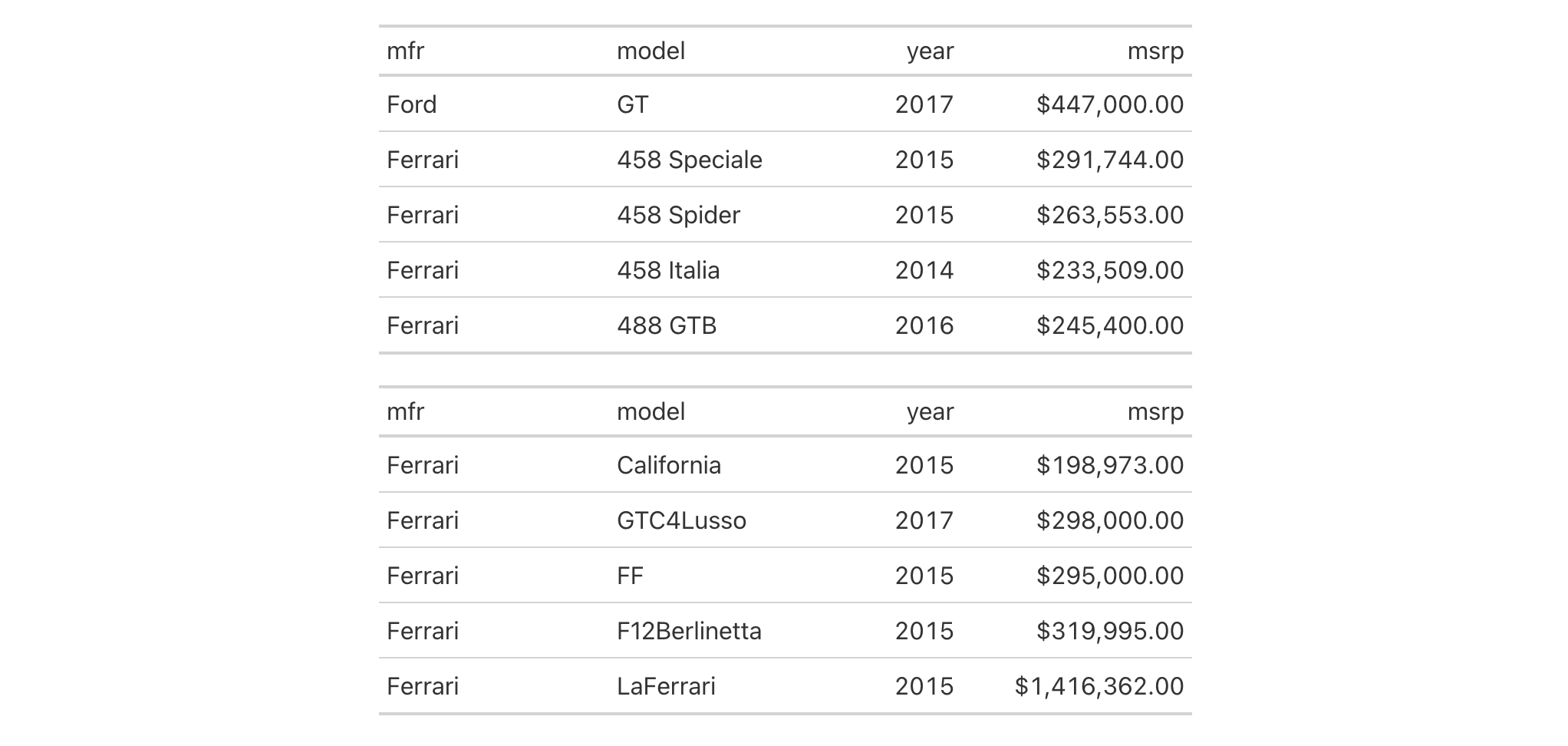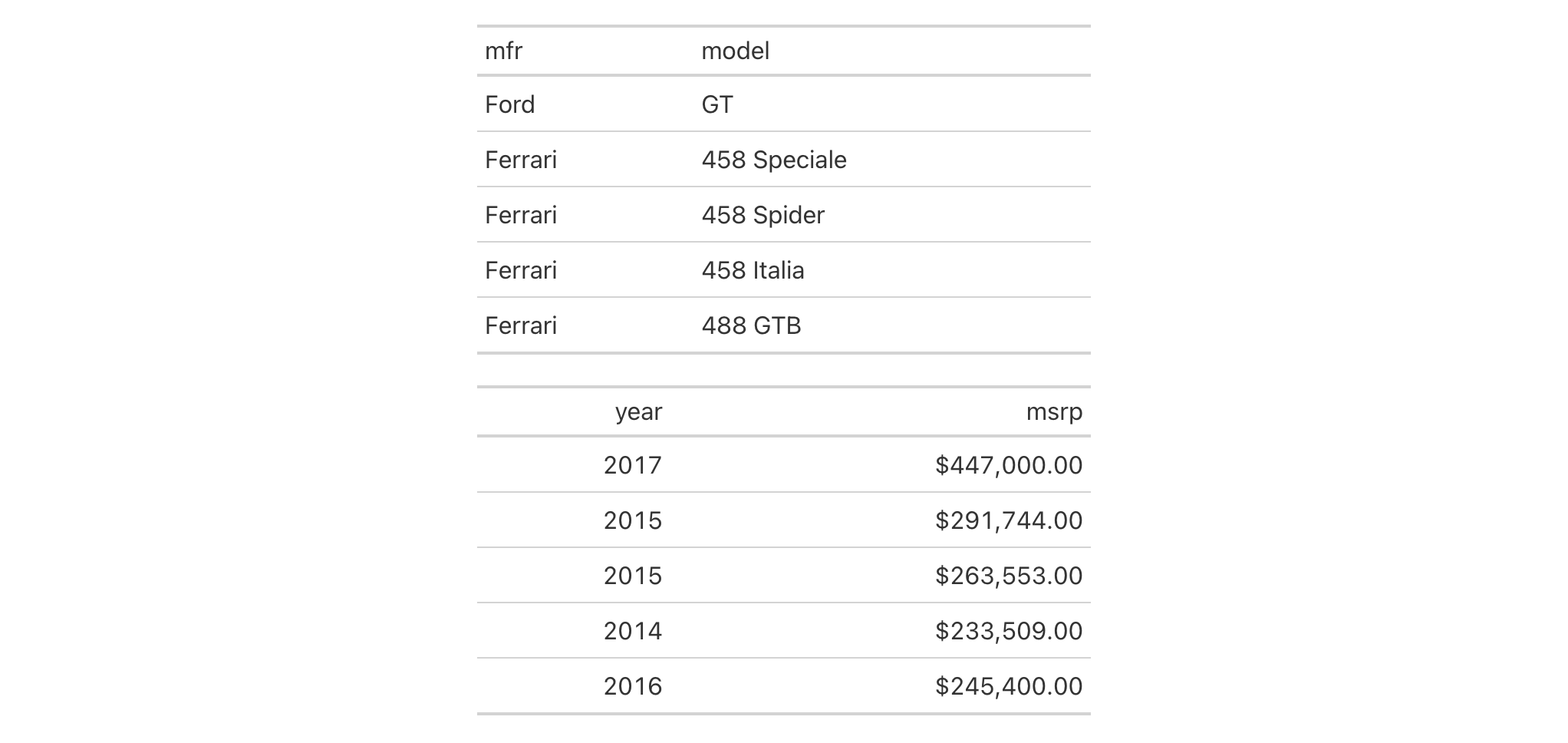With a gt table, you can split it into multiple tables and get that collection in a gt_group object. This function is useful for those cases where you want to section up a table in a specific way and print those smaller tables across multiple pages (in RTF and Word outputs, primarily via gtsave()), or, with breaks between them when the output context is HTML.

## Usage

gt_split(data, row_every_n = NULL, row_slice_i = NULL, col_slice_at = NULL)

## Arguments

data

The gt table data object

obj:<gt_tbl> // required

This is the gt table object that is commonly created through use of the gt() function.

row_every_n

Split at every n rows

scalar<numeric|integer> // default: NULL (optional)

A directive to split at every n number of rows. This argument expects a single numerical value.

row_slice_i

Row-slicing indices

vector<numeric|integer> // default: NULL (optional)

An argument for splitting at specific row indices. Here, we expect either a vector of index values or a function that evaluates to a numeric vector.

col_slice_at

Column-slicing locations

<column-targeting expression> // default: NULL (optional)

Any columns where vertical splitting across should occur. The splits occur to the right of the resolved column names. Can either be a series of column names provided in c(), a vector of column indices, or a select helper function. Examples of select helper functions include starts_with(), ends_with(), contains(), matches(), one_of(), num_range(), and everything().

## Value

An object of class gt_group.

## Examples

Use a subset of the gtcars dataset to create a gt table. Format the msrp column to display numbers as currency values, set column widths with cols_width(), and split the table at every five rows with gt_split(). This creates a gt_group object containing two tables. Printing this object yields two tables separated by a line break.

gtcars |>
dplyr::select(mfr, model, year, msrp) |>
gt() |>
fmt_currency(columns = msrp) |>
cols_width(
year ~ px(80),
everything() ~ px(150)
) |>
gt_split(row_every_n = 5)Use a smaller subset of the gtcars dataset to create a gt table. Format the msrp column to display numbers as currency values, set the table width with tab_options() and split the table at the model column This creates a gt_group object again containing two tables but this time we get a vertical split. Printing this object yields two tables of the same width.

gtcars |>
dplyr::select(mfr, model, year, msrp) |>
gt() |>
fmt_currency(columns = msrp) |>
tab_options(table.width = px(400)) |>
gt_split(col_slice_at = "model")14-2

## Function Introduced

v0.9.0 (Mar 31, 2023)

Other table group functions: grp_add(), grp_clone(), grp_options(), grp_pull(), grp_replace(), grp_rm(), gt_group()